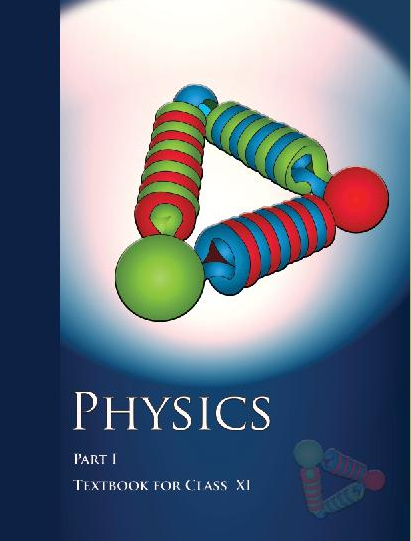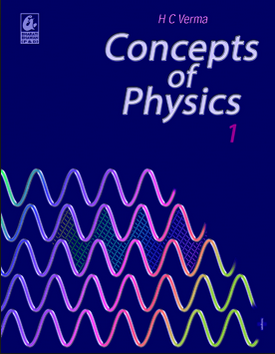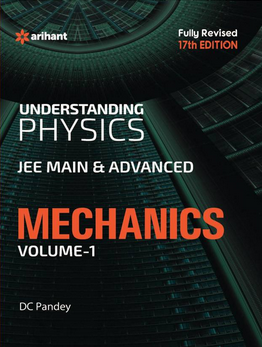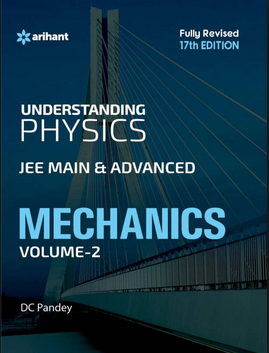# Rotational Motion   Share

## What is Rotational Motion

If a body is pivoted at a point and the force is applied on the body at a suitable point, it rotates the body about the axis passing through the pivoted point. This is the turning effect of the force and the motion of the body is called the Rotational motion.

Rotational Motion

Rotational motion is an integral part of mechanics and the questions asked from this chapter are most difficult. So before starting to solve questions from this chapter, you should have a good hold on concepts from the topics laws of motion, Centre of mass and linear momentum. The questions which are asked from this topic involve conceptual approach rather than the formula-based approach. It is necessary for you to read the theory thoroughly before solving the questions. This article will help you to understand the concepts of rotational motion and how these concepts make complex questions when mixed with the concepts of Newton's basic laws.

## Rotational Motion Topics

Moment of Inertia, Angular Velocity, Torque, Rotation of a rigid body about a fixed axis, Angular Momentum, Conservation of Angular Momentum, Combined Translational and Rotational Motion of a Rigid Body, Uniform Pure Rolling, Instantaneous, Axis of Rotation, Accelerated Pure Rolling, Angular Impulse, Toppling

## Overview of Rotational Motion

In our day to day life, we come across many objects which show rotational motion, the spinning of car wheels, rotation of washing machine agitator, rotation of the earth etc. Before you proceed further in this chapter, you should know what is a rigid body, a rigid body is made of too many particles but the distance between any two particles is always constant. If you remember the concepts of Translatory motion, we consider the rigid body as the point object in which we take the same linear displacement, linear velocity as well as same linear acceleration of all the particles on the rigid body. But in case of rotational motion OR translatory plus rotational motion the particles of the rigid body have different linear displacement, velocity, and acceleration.

Prepare Online for JEE Main/NEET

Crack JEE 2021 with JEE/NEET Online Preparation Program

For most of the students, rotational motion is often the pain in the neck, because it introduces many new terminologies like Moment of Inertia, Torque, and angular momentum etc. But it is not really difficult, because you can relate these terminologies with your previous knowledge of translatory motion. For example just like “centre of mass”, the moment of inertia is also the property of the object that is related to its mass distribution. Same goes with Torque, you can relate it with force.

## When the particle is rotating or rotating plus translating the different particles P1, P2, P3 and P4 have different linear displacement, velocity, and acceleration.## Characteristics of Rotational Motion

In rotational motion, the particles of the rigid body follow a circular path around the rotational axis, the rotational axis could be fixed or it could be unfixed. The example for fixed axis rotational motion is the rotation of a fan, in which each particle on the blade is following a circular path around the axle of the motor of the fan. The example for an unfixed axis of rotational motion is spinning top, in the spinning top, the tip of the top is an unfixed axis around which all the particles are following a circular path.

## How to start preparing for rotational motion?

First of all, learn how to calculate the moment of inertia (MOI) of different objects around different axes. You should also get familiar with using two theorems on MOI, i.e. Theorem of Parallel axes and Theorem of Perpendicular axes. Then only you should go towards calculating other parameters required in the problem. Rotational motion involves a mixture of concepts, hence You need to practice a lot more on this topic. For learning concepts through questions solving, students can take help from our Entrance360 experts. This platform is best for learning because learning concepts through problem-solving help in building concepts rather than solving all questions after reading a full chapter. This can create doubts while solving hence it will result in more confusion and student might get trapped.

Rotational Motion Formulas

## Notes for rotational motion

• Make a plan to prepare for the chapter and Stick to a Timetable. You can make timetable according to available time left for preparation and try to prepare according to it.

• Don’t try to memorize MOI of different objects, rather first calculate it yourself and then memorize it.

• Read carefully on the examples given in NCERT book. Start solving the questions only after you understood all the examples.

• Give mock tests chapter-wise from time to time, this will ensure you have a good hold on concepts.

• Look at the solution/answer only after giving a good number of attempts.

## Books for Rotational Motion

For understanding concepts, students can consider NCERT books. But for question-solving students should consider Understanding Physics by D. C. Pandey (Arihant Publications).

You don't have to study the whole book to understand the concept from this chapter because we will provide you exact page number and line number of these books where you will get these concepts to read.

## Physics Chapter wise Notes For Engineering and Medical Exams

 Chapters No. Chapters Name Chapter 1 Physics and Measurement Chapter 2 Kinematics Chapter 3 Laws of motion Chapter 4 Work Energy and Power Chapter 6 Gravitation Chapter 7 Properties of Solids and Liquids Chapter 8 Kinetic theory of Gases Chapter 9 Thermodynamics Chapter 10 Oscillations and Waves Chapter 11 Electrostatics Chapter 12 Current Electricity Chapter 13 Magnetic Effects of Current and Magnetism Chapter 14 Electromagnetic Induction and Alternating currents Chapter 15 Electromagnetic Waves Chapter 16 Optics Chapter 17 Dual Nature of Matter and Radiation Chapter  18 Atoms And Nuclei Chapter 19 Electronic devices Chapter 20 Communication Systems Chapter 21 Experimental skills

### Topics from Rotational Motion

• Rigid body rotation ( JEE Main, MHT-CET ) (2 concepts)
• Basic concepts of rotation ( JEE Main, MHT-CET ) (4 concepts)
• Moment of Inertia introduction ( JEE Main, MHT-CET ) (6 concepts)
• Values of moments of inertia for simple geometrical objects ( JEE Main, MHT-CET ) (18 concepts)
• Parallel and Perpendicular axes theorems and its applications ( JEE Main, MHT-CET ) (4 concepts)
• Radius of Gyration ( JEE Main, MHT-CET ) (2 concepts)
• Equationof rotational motion ( JEE Main, MHT-CET ) (28 concepts)
• Angular momentum and its applications ( JEE Main, MHT-CET ) (10 concepts)
• Centre of massof two-particle system ( JEE Main, MHT-CET ) (4 concepts)
• Centre of mass of rigid body ( JEE Main, MHT-CET ) (32 concepts)
• Rigid body and its centre of Mass ( JEE Main, MHT-CET ) (26 concepts)
• Basic concepts of rotational motion ( JEE Main, MHT-CET ) (6 concepts)
• Conservation of angular momentum and its applications ( JEE Main, MHT-CET ) (6 concepts)
• Moment of Inertia ( JEE Main, MHT-CET ) (24 concepts)
• Combined rotation and translation motion ( JEE Main, MHT-CET ) (8 concepts)

### Important Books for Rotational Motion

••••Exams
Articles
Questions